• antenna = 1; % 天线数 C = 300000000; % m/s fadc = 10; % M nFrame = 200; % 帧数 chirp = 128; % 一帧的chirp数 point = 256; % 一chirp中的点数 B = 400; % Mhz T = 100; % us PRT = 300;... % 速度分辨率
antenna = 1; % 天线数
C = 300000000; % m/s

nFrame = 200; % 帧数
chirp = 128; % 一帧的chirp数
point = 256; % 一chirp中的点数
B = 400; % Mhz
T = 100; % us
PRT = 300; % us

k = B/T;% Mhz/us 斜率
centerB = 24 + B/(2*1000);% GHz 中心频率

Range_r = C/(2*k*point/(fadc*1000) *1000000000); % 距离分辨率
Doppler_r = C/(2*chirp*antenna*centerB*PRT*1000); % 速度分辨率

展开全文• 目录距离多普勒(Range-Dopple Matrix)处理方法快时间维度处理(Range-FFT)慢时间维度处理(Doppler-FFT)RDM中距离分辨率速度分辨率推导方法参考资料 距离多普勒(Range-Dopple Matrix)处理方法   众所周知，距离...

距离多普勒(Range-Dopple Matrix)处理方法

众所周知，距离多普勒处理方法(Range-Dopple Matrix，简称RDM)是FMCW雷达进行多目标信息提取的有效手段，通过对雷达发送的多个周期的Chirp序列以及回波信息进行快时间维度和慢时间维度的处理，即可得到距离多普勒热力图，进而可以提取多目标的距离和速度信息。

插图来源于参考资料在FMCW的差拍信号中，我们知道，差拍信号的频率为
f m o v i n g B e a t = f s t a t i c B e a t ± f d = 2 f c R C t c ± 2 f v C (1) f_{movingBeat} = f_{staticBeat} \pm f_d = \frac{2f_cR}{Ct_c} \pm \frac{2fv}{C} \tag 1   其中 f m o v i n g B e a t f_{movingBeat} f s t a t i c B e a t f_{staticBeat} 分别为目标运动和静止状态下差拍信号的频率， f d f_d 为多普勒频率， f c f_c 为扫频带宽， R R 为目标距离， C C 为光速， t c t_c 为扫频周期， f f 为Chirp信号中心频率， v v 为目标速度。

快时间维度处理(Range-FFT)

快时间维度即单个周期的Chirp序列扫频周期时间很短，短到几乎可以将多普勒频率带来的影响忽略不计( t c t_c ↓，公式(1)中 f s t a t i c B e a t f_{staticBeat} 项占了主要的位置)，认为此时通过RDM热力图提取到的动目标在距离维度上的动目标差频 f m o v i n g B e a t f_{movingBeat} 与静目标差频 f s t a t i c B e a t f_{staticBeat} 近似相等，即 f m o v i n g B e a t ≈ f s t a t i c B e a t = 2 f c R C t c (2) f_{movingBeat} \approx f_{staticBeat} = \frac{2f_cR}{Ct_c} \tag 2   那么通过快时间维度的每一帧数据，提取频谱峰值对应的横坐标频率，即可对目标的距离进行求解；即 R = C t c 2 f c ⋅ f s t a t i c B e a t (3) R = \frac{Ct_c}{2f_c}\cdot f_{staticBeat} \tag 3   快时间维处理示意图如下

插图来源于参考资料慢时间维度处理(Doppler-FFT)

因为我们知道，在快时间维的处理中，认为速度带来的影响忽略不计，通过对多个Chirp序列进行多帧数据的堆积，此时在第二个维度上(即慢时间维度上，多帧数据对应的同一距离单元上)速度带来的频率影响就不可忽略，此时慢时间维度上求得的频率即为多普勒频率，即 f d = 2 f v C (4) f_d = \frac{2fv}{C} \tag 4   所以有 v = f d C 2 f (5) v = \frac{f_dC}{2f} \tag 5
慢时间维处理示意图如下

插图来源于参考资料慢时间维度的处理是经过多个Chirp序列积累后对同一距离单元进行FFT的结果，故称为慢时间维度，

为什么是同一距离单元？
因为Range-FFT中同一个横坐标对应相同的 f m o v i n g B e a t f_{movingBeat} ，快时间维度下 f m o v i n g B e a t f_{movingBeat} 约等于 f s t a t i c B e a t f_{staticBeat} ，由公式(2)和公式(3)可知，对应同一距离单元

快时间维度和慢时间维度处理总览

插图来源于参考资料经过处理后可得到如下的距离多普勒热力图(Range-Dopple Heat Map)

插图来源于参考资料RDM中距离分辨率和速度分辨率推导方法

网上关于RDM方法中距离分辨率和速度分辨率推导的资料实在太少，几乎都是两个长得不太好看公式直接糊你脸上，我的感受就是老人、地铁、看手机.jpg(此处省略表情包)，于是决定记录下推导过程，正所谓难者不会，会者不难。
首先回顾下数字信号处理中第K个采样点的频率 f k f_k 与采样频率 f s f_s 间的关系，我们知道，第K个采样点的角频率服从如下关系
ω k = k N s ⋅ 2 π = Ω ⋅ T s = 2 π f k ⋅ 1 f s \omega_k = \frac{k}{N_s}\cdot2\pi = \Omega\cdot T_s = 2 \pi f_k\cdot \frac{1}{f_s}
其中 N s N_s 为采样点数， Ω \Omega 为模拟角频率， T s T_s 为采样频率，我们取出等式中的第二项和第四项，有
k N s ⋅ 2 π = 2 π f k ⋅ 1 f s \frac{k}{N_s}\cdot2\pi =2 \pi f_k\cdot \frac{1}{f_s}   可得第K个采样点的频率 f k f_k 与采样频率 f s f_s 间的关系为
f k = k ⋅ f s N s (6) f_k = k\cdot \frac{f_s}{N_s} \tag 6
到此就可以正式展开距离分辨率和速度分辨率的推导方法了，上一部分我们说到快时间维度的Range-FFT和慢时间维度的Doppler-FFT，有两个结论性的公式
f m o v i n g B e a t ≈ f s t a t i c B e a t = 2 f c R C t c (7) f_{movingBeat} \approx f_{staticBeat} = \frac{2f_cR}{Ct_c} \tag 7 f d = 2 f v C (8) f_d = \frac{2fv}{C} \tag 8   假设上一部分中距离多普勒热力图中， n 1 n_1 为Range-FFT(快时间维度)中目标对应的坐标序列号， n 2 n_2 为Doppler-FFT(慢时间维度)中同一目标对应的坐标序列号，则依照公式(6)可得
f m o v i n g B e a t = n 1 N s ⋅ f s (9) f_{movingBeat} = \frac{n_1}{N_s} \cdot f_s \tag 9 f d = n 2 N C h i r p ⋅ 1 t c (10) f_d = \frac{n_2}{N_{Chirp}} \cdot \frac{1}{t_c} \tag {10}   其中 N C h i r p N_{Chirp} 为慢时间维度处理中Chirp序列的积累个数；公式(9)类比公式(6)，比较好理解，公式(10)也是类比公式(6)，只不过此时在慢时间维度上采样总数是积累的Chirp序列的总数，采样频率是每一个Chirp序列扫频周期的倒数，即 1 t c \frac{1}{t_c}
由此以来，分别联立公式(7)和公式(9)，联立公式(8)和公式(10)，可得
2 f c R C t c = n 1 N s ⋅ f s \frac{2f_cR}{Ct_c} = \frac{n_1}{N_s} \cdot f_s 2 f v C = n 2 N C h i r p ⋅ 1 t c \frac{2fv}{C} = \frac{n_2}{N_{Chirp}} \cdot \frac{1}{t_c}   可解得
R = C 2 f C ⋅ t c ⋅ n 1 N s ⋅ f s (11) R = \frac{C}{2f_C}\cdot t_c\cdot \frac{n_1}{N_s}\cdot f_s \tag{11}
v = C 2 f ⋅ n 2 N C h i r p ⋅ 1 t c (12) v = \frac{C}{2f} \cdot \frac{n_2}{N_{Chirp}}\cdot \frac{1}{t_c}\tag{12}   因为
t c = N s ⋅ T s = N s f s (13) t_c = N_s\cdot T_s = \frac{N_s}{f_s} \tag{13} t s e q = N C h i r p ⋅ t c (14) t_{seq} = N_{Chirp}\cdot t_c\tag{14}   将(13)代入(11)，将(14)代入(12)，可得
R = C 2 f c ⋅ n 1 R = \frac{C}{2f_c} \cdot n_1 v = C 2 f t s e q ⋅ n 2 v = \frac{C}{2ft_{seq}} \cdot n_2   此时，就得到了距离分辨率和速度分辨率，分别为
R r e s = C 2 f c R_{res} = \frac{C}{2f_c} v r e s = C 2 f N C h i r p t c = C 2 f t s e q v_{res} = \frac{C}{2fN_{Chirp}t_c} = \frac{C}{2ft_{seq}}

仿真程序代码

仿真程序代码如下，作者：Elias.J

%%%%%%%%%%%%%%%%%%%%%%%%%%%%%%%%%%%%%%%%%%%%%%%%%%%%%%%%%%%%%%%%%%%%%
% File name: RDM.m
% Author: Elias.J@CSDN
% CSDN: https://blog.csdn.net/qq_41248471
%%%%%%%%%%%%%%%%%%%%%%%%%%%%%%%%%%%%%%%%%%%%%%%%%%%%%%%%%%%%%%%%%%%%%
%% Initial operation
close all;
clc;
tarR = [15 25];    %target range
tarV = [-3 10];     %target velocity
c = 3*10^8;
f0 = 24.25*10^9;
T = 0.0002;   %chirp Sweep Time
B = 400*10^6;
L = 128;            %slow-time dimension,num of chirps
N = 128;           %fast-time dimension,num of samples
S1 = zeros(L,N);
for l = 1:L
for n = 1:N
S1(l,n) = 500*exp(1i*2*pi*((2*B*(tarR(1)+tarV(1)*T*l)/(c*T)+(2*f0*tarV(1))/c)*T/N*n+((2*f0)*(tarR(1)+tarV(1)*T*l))/c));
end
end
S1 = awgn(S1,20);

S2 = zeros(L,N);
for l = 1:L
for n = 1:N
S2(l,n) = 500*exp(1i*2*pi*((2*B*(tarR(2)+tarV(2)*T*l)/(c*T)+(2*f0*tarV(2))/c)*T/N*n+((2*f0)*(tarR(2)+tarV(2)*T*l))/c));
end
end
S2 = awgn(S2,20);
%% range fft processing
hanning1 = hanning(N,'periodic');
% hanning1 = ones(N,1);
sigRWin = zeros(L,N);
for ii = 1:L
end
for ii = 1:L
end
%% doppler fft processing
hanning2 = hanning(L,'periodic');
% hanning2 = ones(N,1);
sigDWin(:,ii) = hanning2.*sigRfft(:,ii);
end
end
%% Visualization
xlabel('Range/m'),ylabel('Frame');
figure,mesh(abs(sigRfft)),title('Range-FFT');
xlabel('距离/m'),ylabel('速度/mps');
xlim([0 45]);
ylim([-18 18]);
xlabel('距离/m'),ylabel('速度/mps'),zlabel('幅值/dB');

%% Window Test

hanning1 = ones(N,1);
sigRWin = zeros(L,N);
for ii = 1:L
end
for ii = 1:L
end

hanning2 = ones(N,1);
sigDWin(:,ii) = hanning2.*sigRfft(:,ii);
end
end

figure(1),
xlabel('距离/m'),ylabel('速度/mps');
title('加矩形窗');
figure(2),
xlim([0 45]);
ylim([-18 18]);
xlabel('距离/m'),ylabel('速度/mps'),zlabel('幅值/dB');
title('加矩形窗');

hanning1 = hanning(N,'periodic');
sigRWin = zeros(L,N);
for ii = 1:L
end
for ii = 1:L
end

hanning2 = hanning(L,'periodic');
sigDWin(:,ii) = hanning2.*sigRfft(:,ii);
end
end

figure(1),
xlabel('距离/m'),ylabel('速度/mps');
title('加汉宁窗');
figure(2),
xlim([0 45]);
ylim([-18 18]);
xlabel('距离/m'),ylabel('速度/mps'),zlabel('幅值/dB');
title('加汉宁窗');

hanning1 = hamming(N,'periodic');
sigRWin = zeros(L,N);
for ii = 1:L
end
for ii = 1:L
end

hanning2 = hamming(L,'periodic');
sigDWin(:,ii) = hanning2.*sigRfft(:,ii);
end
end

figure(1),
xlabel('距离/m'),ylabel('速度/mps');
title('加汉明窗');
figure(2),
xlim([0 45]);
ylim([-18 18]);
xlabel('距离/m'),ylabel('速度/mps'),zlabel('幅值/dB');
title('加汉明窗');

hanning1 = blackman(N,'periodic');
sigRWin = zeros(L,N);
for ii = 1:L
end
for ii = 1:L
end

hanning2 = blackman(L,'periodic');
sigDWin(:,ii) = hanning2.*sigRfft(:,ii);
end
end

figure(1),
xlabel('距离/m'),ylabel('速度/mps');
title('加布莱克曼窗');
figure(2),
xlim([0 45]);
ylim([-18 18]);
xlabel('距离/m'),ylabel('速度/mps'),zlabel('幅值/dB');
title('加布莱克曼窗');
%% 2D CA-CFAR
Pfa = 10^(-6);
Rres_rdm = c/(2*B);
Vres_rdm = c/(2*24.25*10^9*T*L);
Range_Dim = Rres_rdm*[1:N];
Velocity_Dim = Vres_rdm*([1:L] - L/2);
Range_Dopple_Map = abs(sigDfft);

handleWindow_r = 9;
handleWindow_c = 9;
handleWindow = zeros(handleWindow_r,handleWindow_c);
proCell_r = 5;
proCell_c = 5;
proCell = zeros(proCell_r,proCell_c);
[r c] = size(Range_Dopple_Map);
CFAR_Map_r = r - (handleWindow_r-1);
CFAR_Map_c = c - (handleWindow_c-1);
CFAR_Map = zeros(CFAR_Map_r,CFAR_Map_c);
referCellNum = handleWindow_r*handleWindow_c - proCell_r*proCell_c;
alpha = referCellNum*(Pfa^(-1/referCellNum) - 1);
for i = 1:CFAR_Map_r
for j = 1:CFAR_Map_c
handleWindow = Range_Dopple_Map(i:i+handleWindow_r-1,j:j+handleWindow_c-1);
proCell = handleWindow(1+(handleWindow_r - proCell_r)/2:handleWindow_r - (handleWindow_r - proCell_r)/2,1+(handleWindow_c - proCell_c)/2:handleWindow_c - (handleWindow_c - proCell_c)/2);
Beta = (sum(sum(handleWindow)) - sum(sum(proCell)))/(referCellNum);
CFAR_Map(i,j) = alpha*Beta;
end
end

CFAR_MapRange_Dim = Range_Dim(1 + handleWindow_r - proCell_r:N - (handleWindow_r - proCell_r));
CFAR_MapVelocity_Dim = Velocity_Dim(1 + handleWindow_c - proCell_c:N - (handleWindow_c - proCell_c));
figure,subplot(121);
mesh(Range_Dim,Velocity_Dim,10*log10(Range_Dopple_Map));
xlabel('距离/m'),ylabel('速度/mps'),zlabel('幅值/dB');
title('2D-FFT')
subplot(122);
mesh(CFAR_MapRange_Dim,CFAR_MapVelocity_Dim,10*log10(CFAR_Map));
xlabel('距离/m'),ylabel('速度/mps'),zlabel('幅值/dB');
title('2D-CFAR检测判决门限')

figure,subplot(121);
image(Range_Dim,Velocity_Dim,10*log10(Range_Dopple_Map));
xlabel('距离/m'),ylabel('速度/mps');
title('距离多普勒图');
subplot(122);
image(CFAR_MapRange_Dim,CFAR_MapVelocity_Dim,10*log10(CFAR_Map));
xlabel('距离/m'),ylabel('速度/mps');
title('2D-CFAR检测判决门限')

CRange_Dopple_Map = Range_Dopple_Map(1 + handleWindow_r - proCell_r:N - (handleWindow_r - proCell_r),1 + handleWindow_c - proCell_c:N - (handleWindow_c - proCell_c));
[comR comC] = find(CRange_Dopple_Map < CFAR_Map);
for i = 1:length(comR)
CRange_Dopple_Map(comR(i),comC(i)) = 0;
end

figure,mesh(CFAR_MapRange_Dim,CFAR_MapVelocity_Dim,CRange_Dopple_Map);
xlabel('距离/m'),ylabel('速度/mps'),zlabel('幅值');
figure,image(CFAR_MapRange_Dim,CFAR_MapVelocity_Dim,CRange_Dopple_Map);
xlabel('距离/m'),ylabel('速度/mps');

参考资料

展开全文信号处理
• 分析了出瞳扫描方式扫描视场角与激光雷达光学系统参数的关系，认为最大扫描现场角比主天线望远镇...在信号过程线性的前提下，根据强度像的瑞利判据提出了扫描激光雷达分辨率公式建议。对主要结论都进行了实验验证。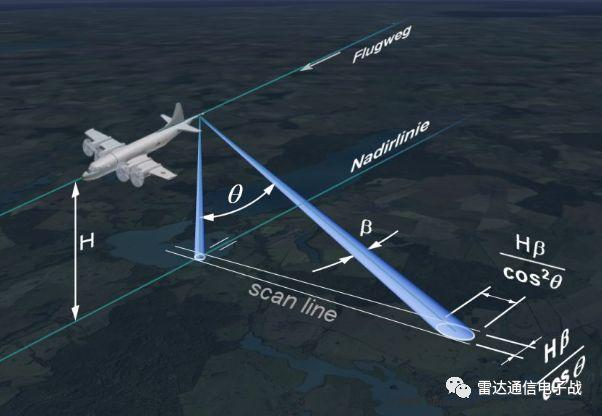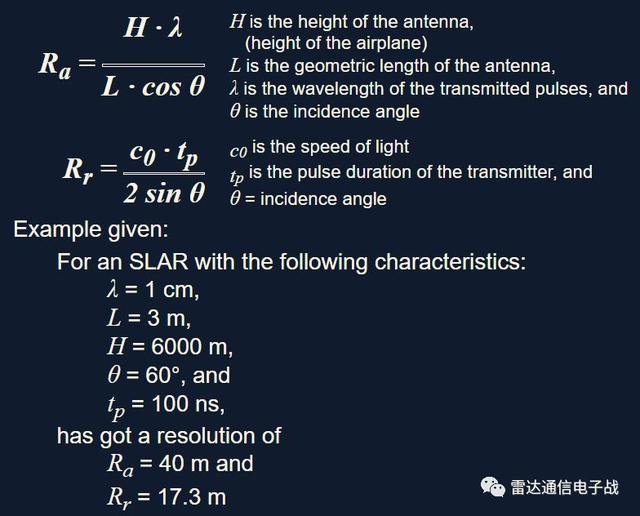合成孔径雷达(SAR)思想的产生

合成孔径的概念始于50年代初期。当时，有些科学家想突破经典分辨率的限制，提出了一些新的设想：

• 利用目标与雷达的相对运动所产生的多普勒频移现象来提高分辨力；
• 用线阵天线概念证明运动着的小天线可获得高分辨力。

SAR基本概念

合成孔径雷达天线往往仅用单个辐射单元，沿一直线依次在若干个位置平移，且在每一个位置发射一个脉冲信号，接收相应发射位置的雷达回波信号并储存起来，然后通过信号处理的方法产生一个等效的长的线性阵列天线。合成孔径雷达的特点是分辨率高，能全天候工作，可有效地识别伪装和穿透掩盖物。

相参性

要想通过在不同的位置发射信号并收集后再联合处理，那么首先就要确保发射的脉冲是相参的，相参性是SAR系统获得高分辨率的必要条件。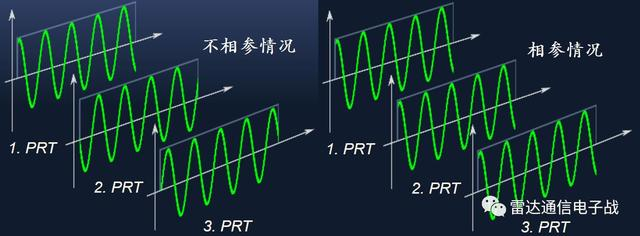发射信号、本振电压、相参震荡电压和定时器的触发脉冲均由同一基准信号提供，接收机也需要具备很高的时间精度。

多普勒历程

随着平台的运动，地面目标逐渐进入雷达波束，平台接近目标时多普勒频率为正，远离目标时为负，频率随时间变化曲线的斜率为负，目标的多普勒历程如下图所示。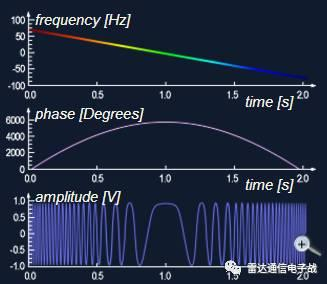SAR的距离和方位分辨率

SAR通过脉冲压缩技术改善距离分辨率，它与发射信号的带宽有关，带宽越大，分辨率越小；通过合成孔径技术改善方位分辨力，条带SAR理论上可以达到天线尺寸的1/2，聚束SAR分辨率更小。

高的分辨力要求采用小的天线而不是大的天线，并且与距离和波长无关。当然，受到其他因素的影响，天线孔径也不可能无限小。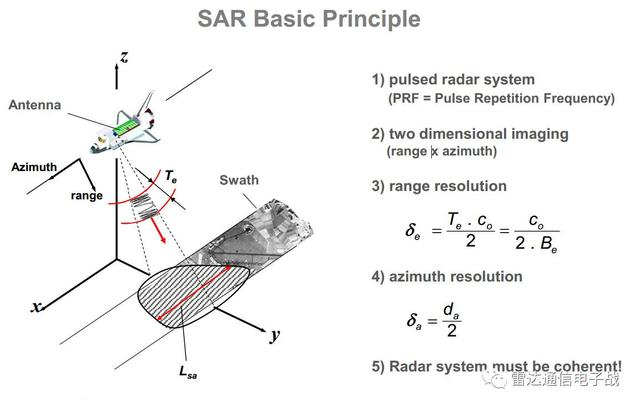回波的存储

SAR是需要存储雷达回波，由于数据不是同时采集的，需要对一定的时间间隔内接收的信号进行运算。 A/D转换之后对数字信号进行存储，选择存储介质必须考虑到信息记录的速率、记录的数据容量、完成方位压缩和脉冲压缩时存储数据的读取速度。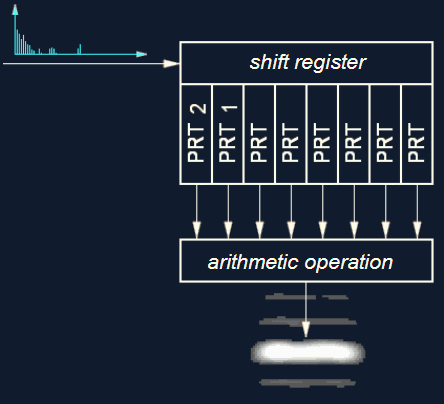SAR的信号模型和处理过程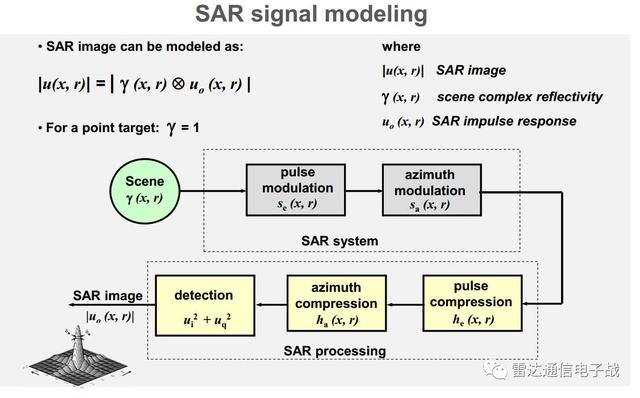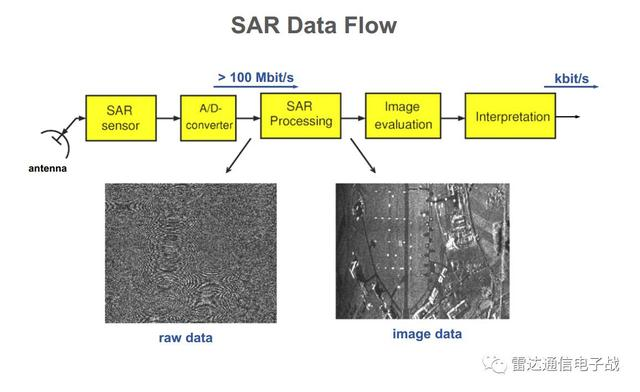SAR天线在每个位置发射脉冲信号并接收目标回波并按顺序存储，然后通过二维匹配滤波实现目标的距离和方位向的高分辨。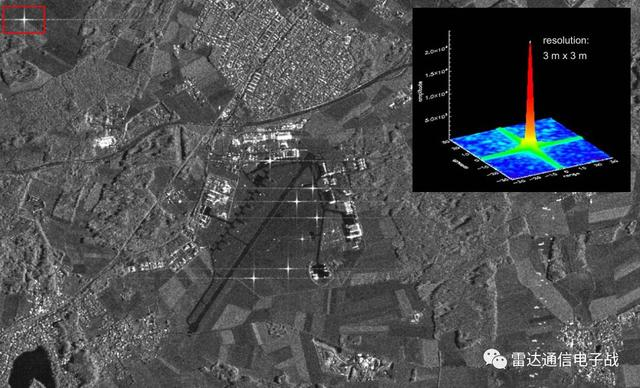运动补偿

SAR信号处理是假定雷达随飞机做直线等速飞行。实际上，运载天线的飞行器总是与这种典型的直线等速飞行状态有偏差的。因此就需要用辅助设备来补偿非直线运动。

运动补偿设备必须包含能检测飞行路线与直线路径偏离的传感器，可以用各种方式使用此敏感元件的输出。为了完善运动补偿，还必须调整接收信号的相位，以补偿实际天线与理想的形成合成天线位置之间的偏移。

极化合成孔径雷达

极化是电磁波的本质属性之一，是除频率、幅度、相位之外的又一维重要信息。电磁波的传播和散射都是矢量现象，而极化正是用来研究电磁波的这种矢量特征。SAR系统常用四种极化方式——HH、VV、HV、VH。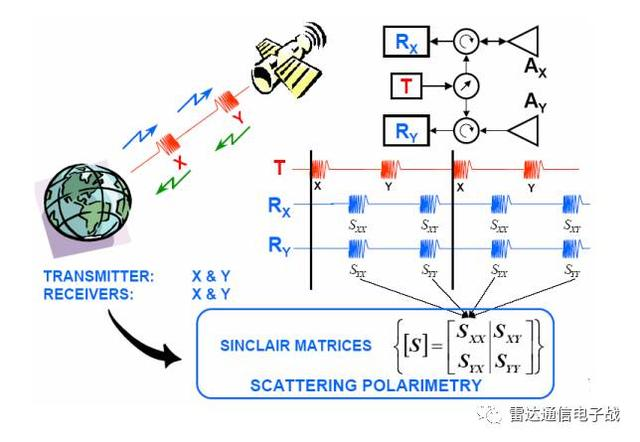雷达发射的能量脉冲的电场矢量，可以在垂直或水平面内被偏振。无论哪个波长，雷达信号可以传送水平(H)或者垂直(V)电场矢量，接收水平(H)或者垂直(V)或者两者的返回信号。

单极化是指(HH)或者(VV)，就是水平发射水平接收或垂直发射垂直接收。气象雷达领域那一般都是(HH)。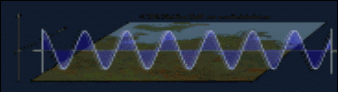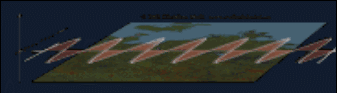双极化是指在一种极化模式的同时，加上了另一种极化模式，如(HH：水平发射水平接收)和(HV：水平发射垂直接收)。全极化技术难度最高，要求同时发射H和V，也就是HH/HV/VV/VH四种极化方式。

电磁波的极化对目标的介电常数、物理特性、几何形状和取向等比较敏感，因而极化测量可以大大提高成像雷达对目标各种信息的获取能力。下图是同一个地区不同极化方式下的成像结果。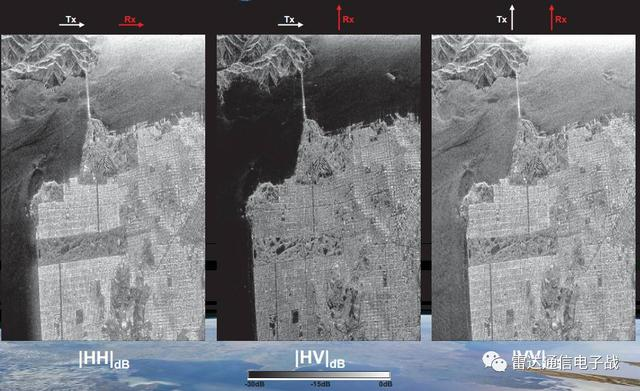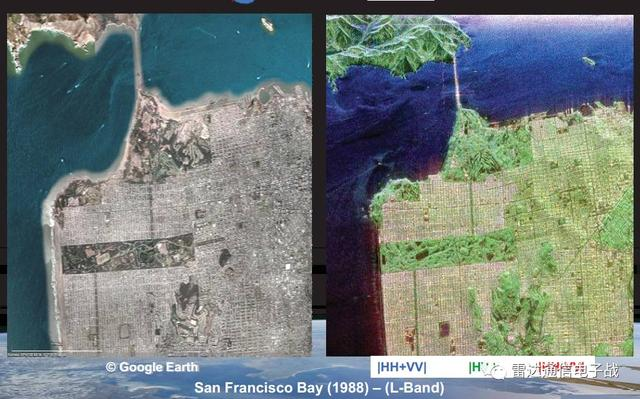雷达极化已经发展成为一种比较成熟的技术，在农业(分辨不同的农作物耕地)、森林(植被高度、衰减系数等生物量的估计、物种识别)、地质(地质结构描述)、水文(表面粗糙度和土壤湿度估计、雪湿度估计)、海冰监测(冰龄和厚度估计)和海洋学(波特性估计，热和波前探测)等很大范围内都得到广泛的研究和应用。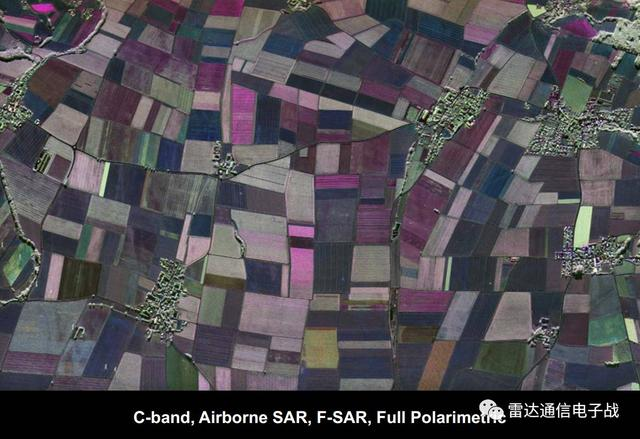水平极化波和垂直极化波在地物或海洋的后向散射系数和相位特性均不相同，因此除了通过多波段来增加遥感的信息含量，也可以通过不同的极化来提高目标的识别的准确度。

通过对雷达目标和地物杂波的极化特性测量与分析，可以实现对不同目标的分类与识别，这在雷达抗干扰领域的作用也日渐突出。

SAR波段选择

雷达波段的选择可以说是相当重要，对于星载SAR，波段选择主要考虑了大气传输窗口、频率和极化对信息提取的影响，图像质量与设备复杂度之间的权衡等因素。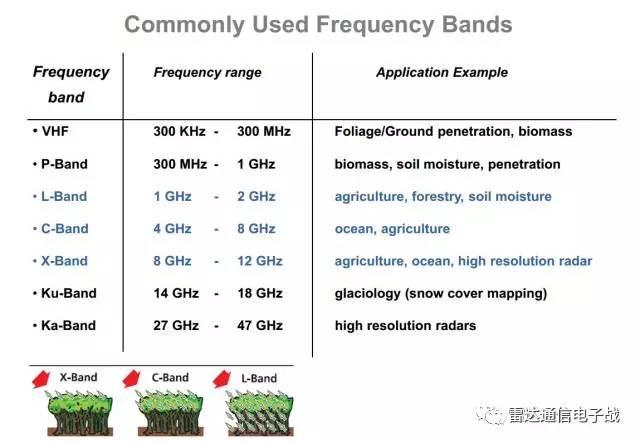大气窗口都知道，大气中的氧和水分子、云雾和雨雹等对高频电磁能量吸收明显，在几个频率上有尖锐的吸收峰值，并且雷达信号在穿透电离层和对流层时会产生相位失真、极化旋转和损耗等，从而使图像出现误差、甚至难以成像。

1GHz频率以下，虽然大气对电磁波的吸收不明显，但是存在明显的极化旋转效应，因此星载SAR的工作频段适宜选择L、C、X波段。

星载SAR观测的后向散射波既包含目标表面发射波，还包含穿透得到的回波。波长越长、穿透能力越强，这种穿透作用在稠密作物或树木的情况下特别明显，从而产生多路径反射，从而形成了极化旋转。

X波段适合对冰的观测和分类，以及对海面污染情况的观察；L波段适合对淡水和穿透地下目标的观测；C波段适合观察海洋上的强目标。

SAR应用领域

合成孔径雷达主要用于航空测量、航空遥感、卫星海洋观测、航天侦察、图像匹配制导等。它能发现隐蔽和伪装的目标，如识别伪装的导弹地下发射井、识别云雾笼罩地区的地面目标等。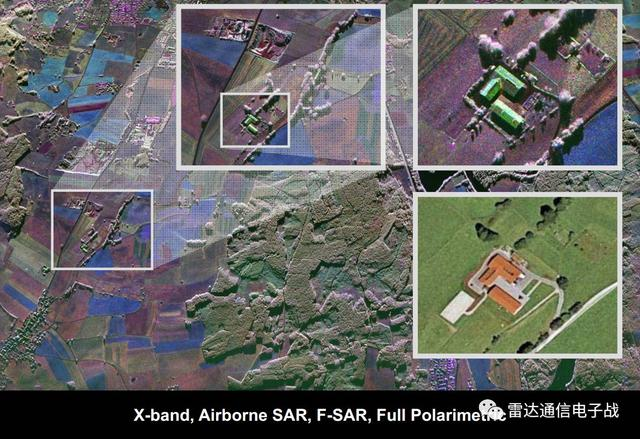展开全文• 根据TI公司那位经典印度老哥的视频，距离分辨率可以如此计算： 假设FMCW的Slope为 SSS，那么在一个chirp内接收到的两个相差时间为 δt\delta tδt的信号所对应的频率可以写为 δt⋅S=δB\delta t \cdot S = \delta B...

以FMCW体制的雷达为例

一、从原始信号处理的角度出发
根据TI公司那位经典印度老哥的视频，距离分辨率可以如此计算：

假设FMCW的Slope为 S S ，那么在一个chirp内接收到的两个相差时间为 δ t \delta t 的信号所对应的频率可以写为

δ t ⋅ S = δ B \delta t \cdot S = \delta B

这样问题就转化成了频率区分的问题，于是

δ t ⋅ S = δ B ≥ f m i n = 1 / T \delta t \cdot S = \delta B \geq f_{min}=1/T ，其中 T T 为观察时长，即ChirpDuration。

如此：

δ d = c / 2 ⋅ δ t ≥ c / ( 2 B ) \delta d =c/2 \cdot \delta t \geq c/(2B) （当然同时还需要考虑本身的快时间采样率上限）。

二、从匹配滤波器输出的角度出发（？）
匹配滤波器输出的峰值信号具有一定程度的展宽，通常用瑞利宽度 β r \beta_r 衡量。如果需要区分两个不同的峰值信号，需要其峰值间隔 δ t \delta t 满足如下要求

δ t ≥ β r \delta t \geq \beta_r ，其中 β r = 1 / B \beta_r=1/B

于是带入距离的计算公式可得相同的结果，

δ d = c / 2 ⋅ δ t ≥ c / ( 2 B ) \delta d =c/2 \cdot \delta t \geq c/(2B)

三、从相干时间的角度出发
对于一个带宽为 B B 的信道，可以认为其相干时间 T c = 1 / B T_c=1/B ，间隔超过相干时间外的多个信号不相干，即可以区分。于是

δ d = c / 2 ⋅ T c ≥ c / ( 2 B ) \delta d =c/2 \cdot T_c \geq c/(2B)

展开全文• 而B_sweep的计算公式为： delta_r为距离分辨率 Doppler Estimation Doppler effect 雷达速度估计是基于一种古老的现象, 叫做多普勒效应. 多普勒效应是波源和观察者有相对运动时, 观察者接受到波的频率与波源发出...
• 毫米波雷达作为辅助驾驶的眼睛，被大量使用在汽车的ACC自适应巡航系统、FCW前方碰撞预警系统以及无人驾驶系统等车载系统中。那么什么是毫米波雷达？毫米波雷达的测量原理又是什么？毫米波雷达，顾名思义，就是工作在...
• 测量目标距离仍然是大多数雷达系统的基本用途。然而，雷达系统在其组成方式、使用的信号、可捕获的信息以及如何在不同的应用中使用这些信息等方面都有了显著的发展。雷达广泛应用于军事和民用领域，包括：监视(威胁...
• 今天进入第11章-脉冲雷达的工作机理。看看脉冲体制的雷达是如何工作的。本章目录如下：11. 脉冲雷达的工作机理11.1脉冲发射的优点11.2脉冲波形11.2.1载波频率11.2.2脉冲宽度11.2.3脉内调制11.2.4脉冲重复频率11.3...
• 点数N：单线雷达一般是一个电机转动获得反射距离，离散采样（与电机速度雷达设计有关）点数一般是固定的，或者几种可选 角分辨率angle_increment：由激光厂商决定，大部分是固定分辨率，比如点数1800个点，360度，...c++
• 脉冲发射的优点：脉冲工作方式可以避免发射机干扰接收机电噪声是机载雷达的难题。发射机不可避免的产生电噪声，并调制发射机的输出信号。噪声产生的边带覆盖了发射频率两侧很宽的频带。虽然噪声边带的功率可以无限小...
• 激光雷达输出的图像也被称为“点云”图像，相邻两个点之间的夹角就是角分辨率。...采样率可以通过角分辨率和帧率计算： 角分辨率0.08°时，每一帧的点云数目：360°/0.08°= 4500； 每秒10帧，则每秒.自动驾驶
• 余杨，眭晓林固体激光技术重点实验室摘要：激光雷达具有抗干扰能力强、分辨率高、隐蔽性好等优点，已被广泛应用于精密测量、侦察监视、火控、制导等领域。针对远程激光测速测距中回波信号微弱难以检测的现实情况，...
• 雷达式测流产品，采用多普勒雷达测速原理，对水流的...另外，监测系统或单位可根据探测获得的速度值（多点测量），加之河道断面几何形状信息等，对天然河流/城市河流、渠/涵/管道剖面水流量进行测定。产品简介DX-...
• 这篇文章的前提条件是在大家已经清楚整个雷达仿真的基本原理并且能自己熟练的根据公式写出雷达信号仿真的距离和相位变化信息。如果这里概念不清楚，请看这qwe14789cn：雷达信号仿真的基本原理——时延与相位的变化​...
• 但是，根据雷达模糊原理可知，我们无法在不断提高的距离分辨率的同时，提高速度分辨率，其中一种分辨率的提高必然会导致另一种分辨率的衰减。我们用时宽带宽积，即时宽与带宽的乘积(TB)表示信号的距离分辨率速度...数字信号处理
• 初识合成孔径雷达SAR

千次阅读 2020-12-22 07:07:57
一个小天线通过“运动”方式就合成一个等效“大天线”，这样可以得到较高的方位向分辨率，同时方位向分辨率与距离无关，这样SAR就可以安装在卫星平台上而可以获取较高分辨率的SAR图像。图1SAR成像原理示意图1、几个...
• 雷达通信电子战，专业知识服务！雷达通信电子战定位于基础知识科普和前沿技术跟踪，专注于资料的搜集和整理分类。发送“0502”获取今日分享资料。节日专属，限时招收：5.1~5.5限时招收《永久铁杆会员》30名雷达用途...
• 利用三维空间平滑和双重线性插值对MUSIC方法进行了改进，给出了分布式雷达超分辨成像的最小信噪比显式表达及其快速计算公式，并据此后分析超分辨成像性能。仿真结果验证了这种基于收发分放置形式的MUSIC超分辨成像...
• 研究了条纹管激光雷达回波信号统计模型，推导出了条纹管成像激光雷达距离分辨率的fCRLB计算公式，分析了fCRLB随距离间隔的变化关系，利用fCRLB对条纹管激光雷达的距离分辨率的影响因素进行了研究。结果表明，距离...
• SAR的计算公式

2020-12-22 07:06:19
我在网上查到三种说法每种都不一样,越看越糊涂1、公式:SARt=SARt-1+AF*(EP-SARt-1)其中AF为加速因子(accelerationfactor),EP为极值(extremeprice)SAR的设计，是每天用与极值差距的某一...我在网上查到三种说法每种都...
• 雷达天线孔径与分辨率

万次阅读 2019-06-03 11:13:52
既然上面提到了分辨率，我们就来看看真实孔径雷达分辨率，主要分距离向分辨率和方位向分辨率。 距离向就是指发射和接收电磁波的方向，距离向的分辨率主要受脉冲信号的宽度 τ 影响，假设一束方波发射出去，遇到...
• 本发明涉及一种雷达分辨率测量方法，具体涉及一种对成像卫星在地球表面成像性能中分辨率的测量方法，属于遥感领域。背景技术：雷达分辨率是指雷达能够分辨不同目标的之间的最小距离，表现了雷达探测目标物体的能力，...
• 距离分辨率表示为△R，是描述雷达将距离非常接近的目标检测为不同目标的能力。雷达系统工作在最小距离Rmin和Rmax之间。Rmin和Rmax之间的距离划分为M个距离单元（门），每个门的宽度为△R。 有多少个距离门？？可以...经验分享
• 雷达回波信号在时间上无法区分时，通过脉冲压缩，可以提高距离分辨率ad bc...